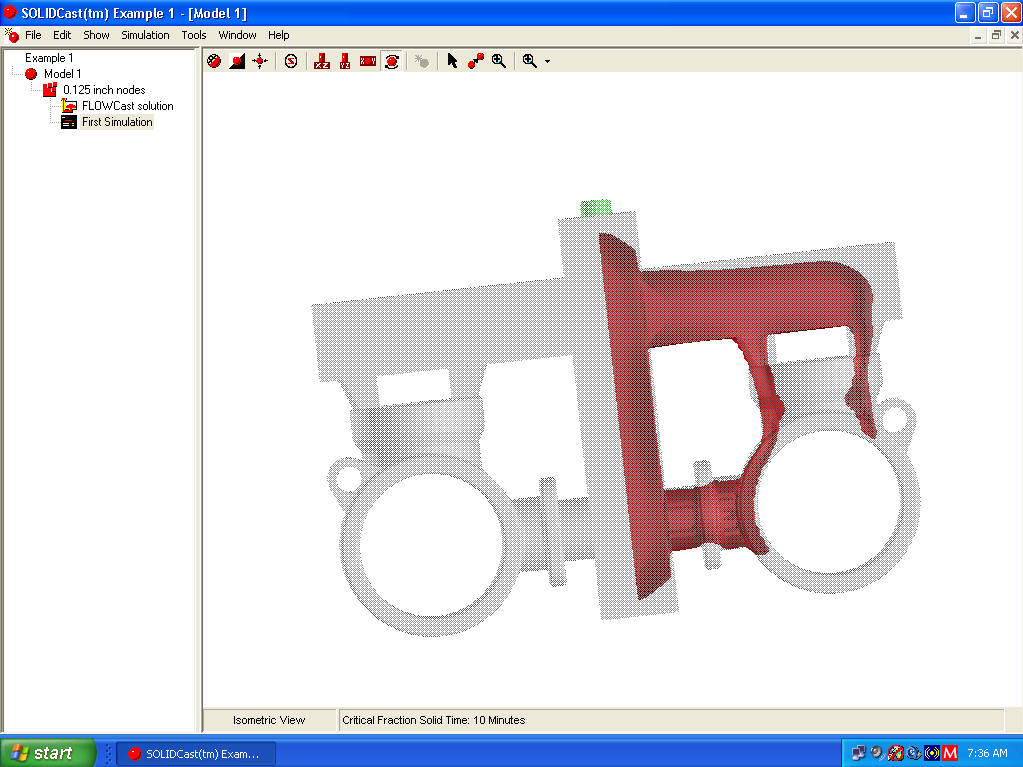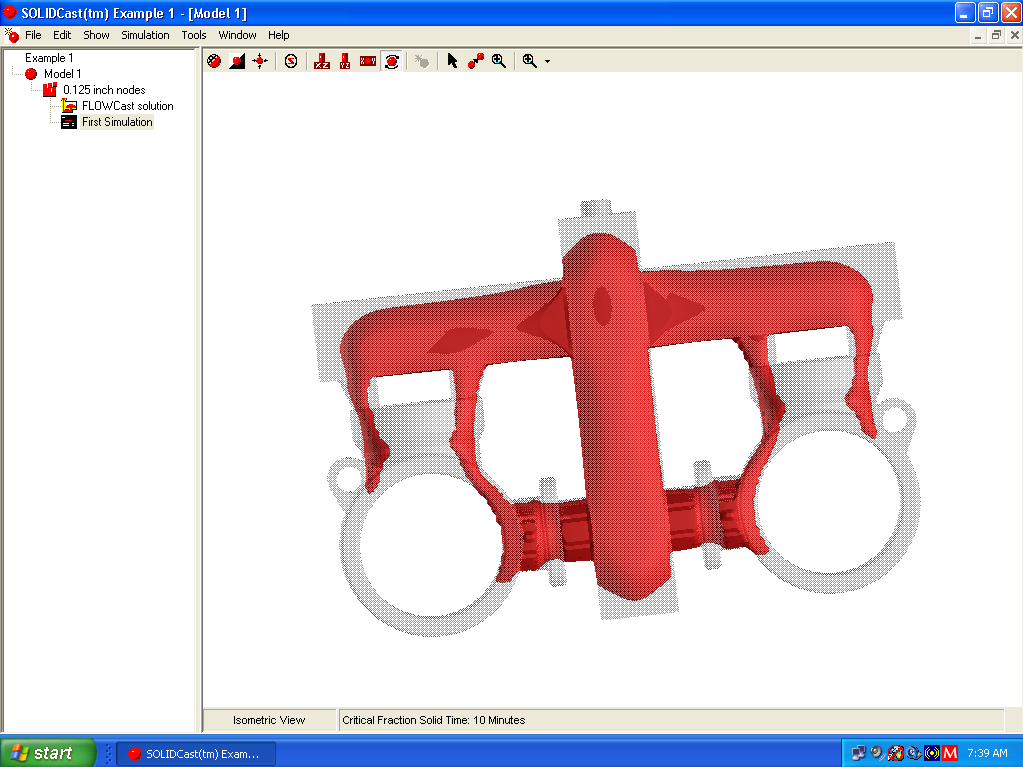# UNIT 39: Mirroring Results

For casting models that are symmetrical, SOLIDCast allows you to specify one or more Planes of Symmetry. This means that a simulation is run on only a portion of the model, which saves time or allows more nodes to be concentrated into a smaller portion of the model (see the section on using Planes of Symmetry). When results are plotted in a model where a Plane of Symmetry has been used, the results are shown only in the section of the model where the model was meshed. As an example, the model below was meshed and simulated with a Lower-X Plane of Symmetry at the center of the model. When Critical Fraction Solid Time is plotted as an Iso-Surface, the result appears as follows:The Mirror function allows you to mirror the results to the other portions of the model, after the simulation has completed. In this way, you can view results as though the entire model had been simulated.

To mirror the results of a simulation, you must have one or more Planes of Symmetry that are NOT ROTATED (i.e., not turned through an angle). If one or more Planes of Symmetry are rotated to any angle other than 0 degrees, mirroring will not work.

To mirror the results, double-click the Simulation icon on the Project Tree, and then close the Simulation Status window. From the menu, select Simulation… Mirror Results. A warning box will appear which tells you that you should be sure that the simulation is complete before performing the mirroring operation. Click OK to proceed. A mirror button will appear briefly on the taskbar. When this disappears, the system has copied the data to the full model.

After the mirroring operation is complete, double-click the simulation icon again and then close the Simulation Status window, then plot results as you would normally.

Mirroring results is a one-time, permanent operation. After performing this operation once for a simulation, the simulation result files are altered and you do not need to perform it again, even if you exit the program and later reload the project. If you try to re-mirror, the system will let you know that this process has already been completed.

In the example model, performing a Mirror function and then plotting Critical Fraction Solid Time shows the following result:Mirroring affects ALL types of output plots, such as Iso-Surface, Cut Plane, CastPic and all movie plots.

If you have two active Planes of Symmetry (such as a Lower X and a Lower Y) and have simulated one-quarter of the casting, the Mirror function will mirror about BOTH planes of symmetry and allow you to plot data within the entire casting.